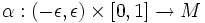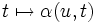# Geodesic variation

Let$M$ be a Riemannian manifold.
A geodesic variation is a variation$\alpha:(-\epsilon,\epsilon) \times [0,1] \to M$ such that for any$u$, the path$t \mapsto \alpha(u,t)$ is a geodesic.